# RD Sharma Solutions for Class 6 Chapter 6: Fractions Exercise 6.7

### RD Sharma Class 6 Solutions Chapter 6 Ex 6.7 PDF Free Download

We at BYJU’S prepare the RD Sharma solutions Class 6 with the key objective of helping students maintain a good academic score. The step by step solutions contain explanations in an interactive manner with the aim of making the subject easy for students. Exercise 6.7 covers important topics like unlike and like fractions and steps followed on comparing and ordering them. The students obtain an overall idea about the concepts and important topics from the exam point of view. To get a better idea about the concepts, the students can download RD Sharma Solutions for Class 6 Maths Chapter 6 Fractions Exercise 6.7 here.

## RD Sharma Solutions for Class 6 Chapter 6: Fractions Exercise 6.7 Download PDF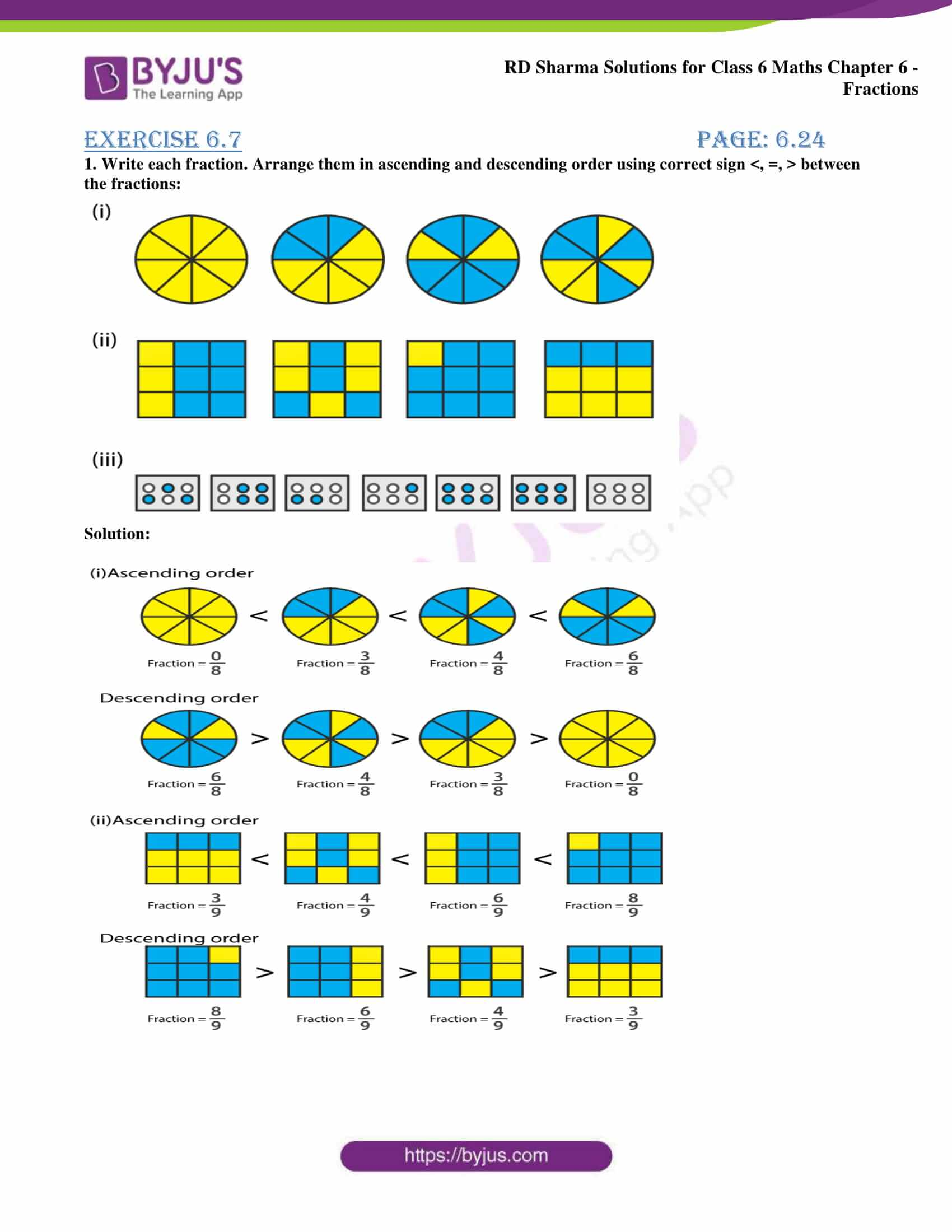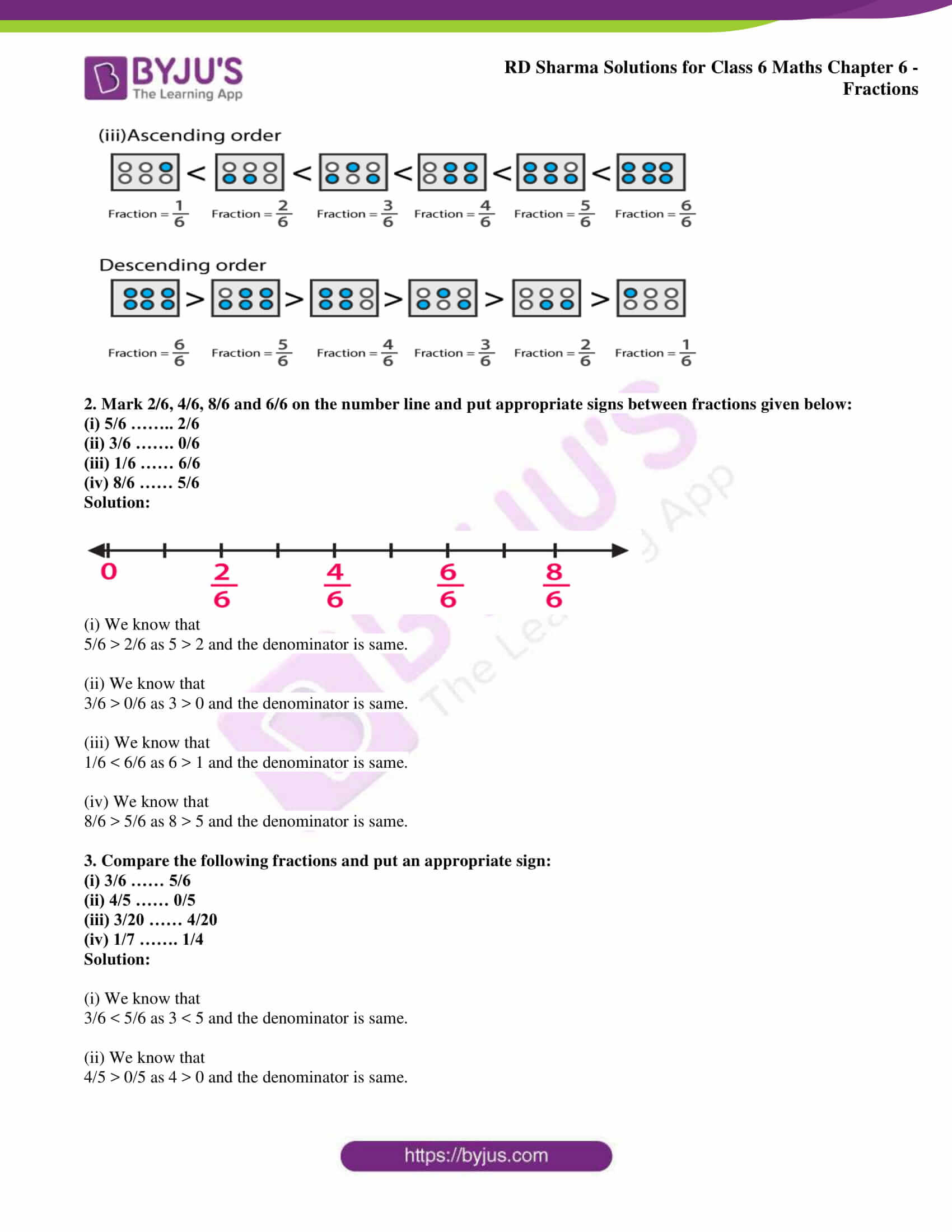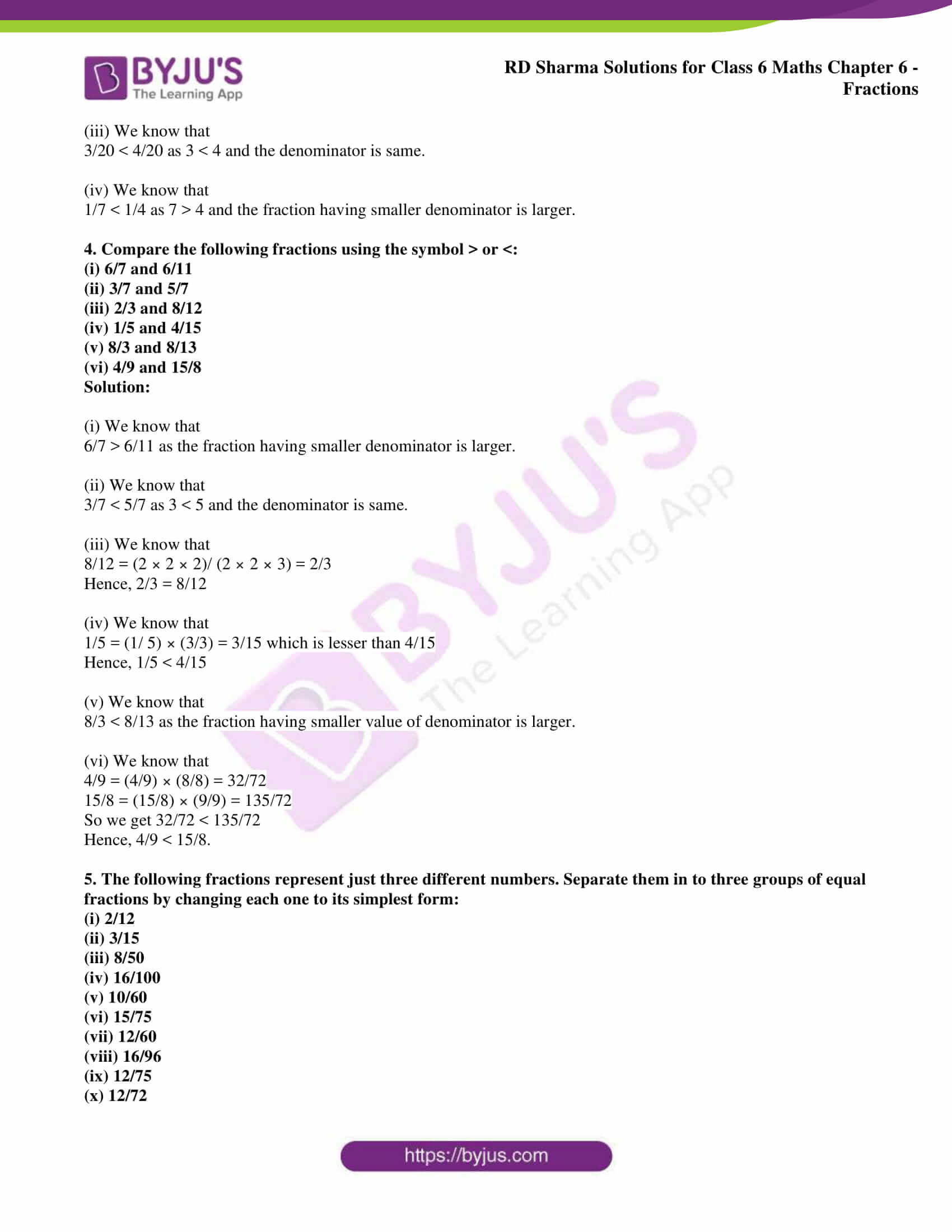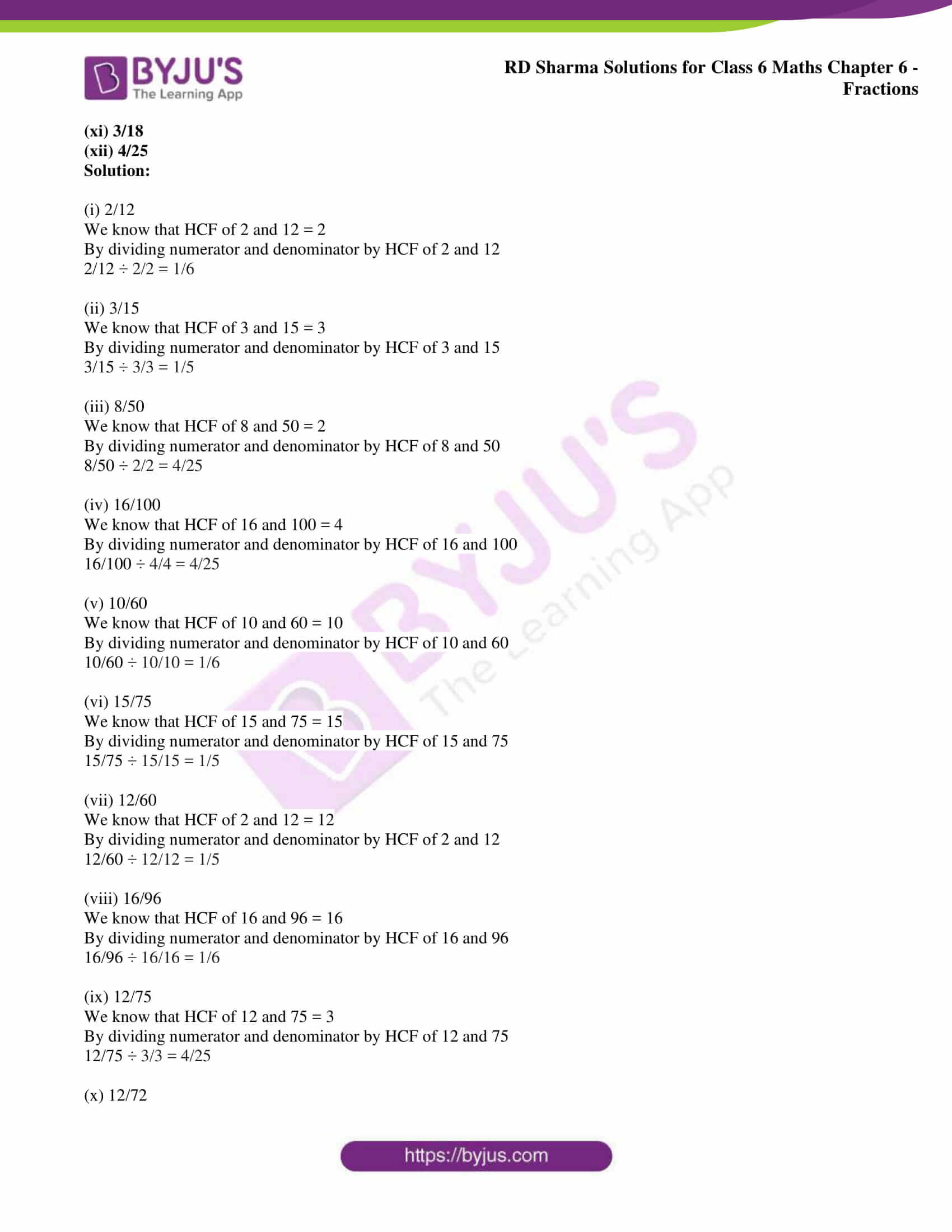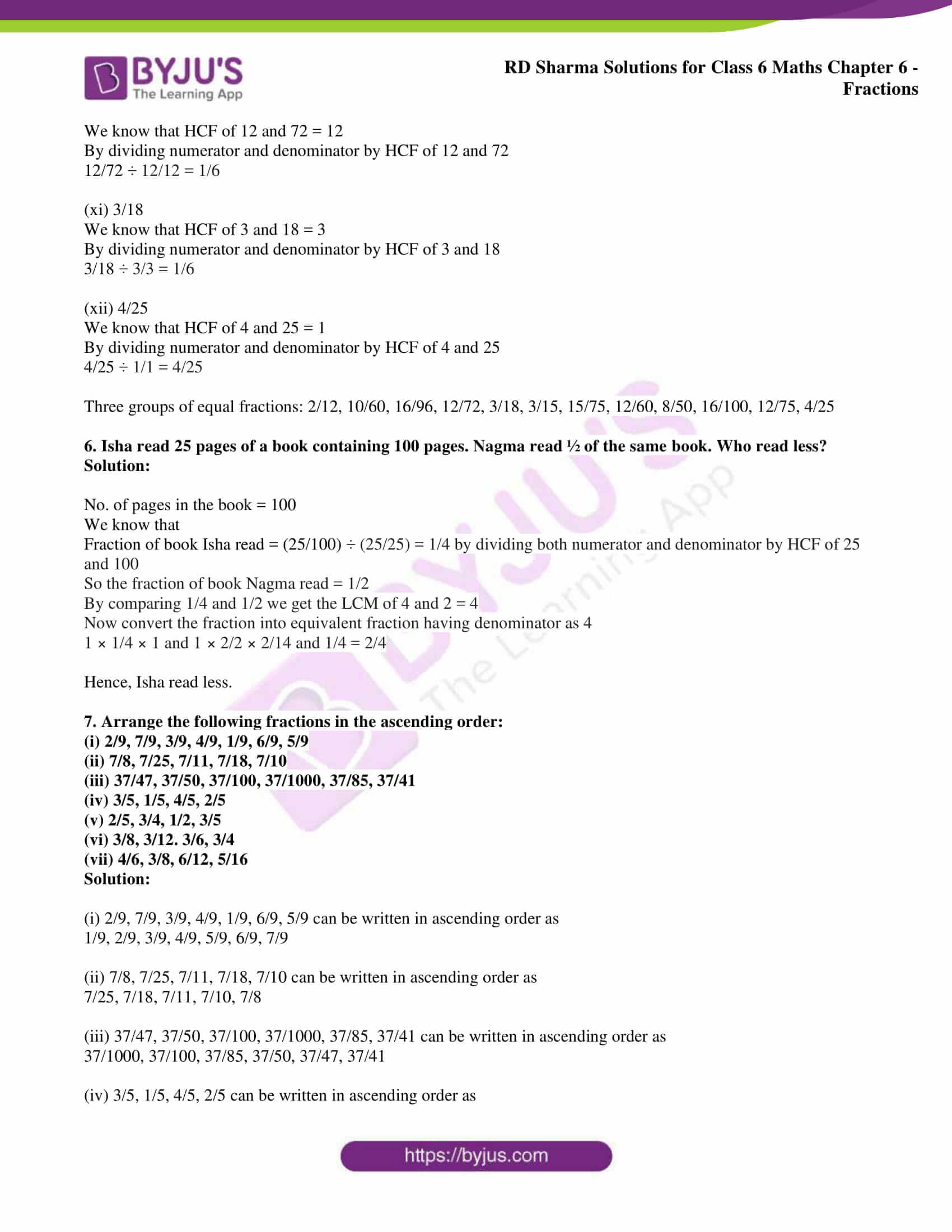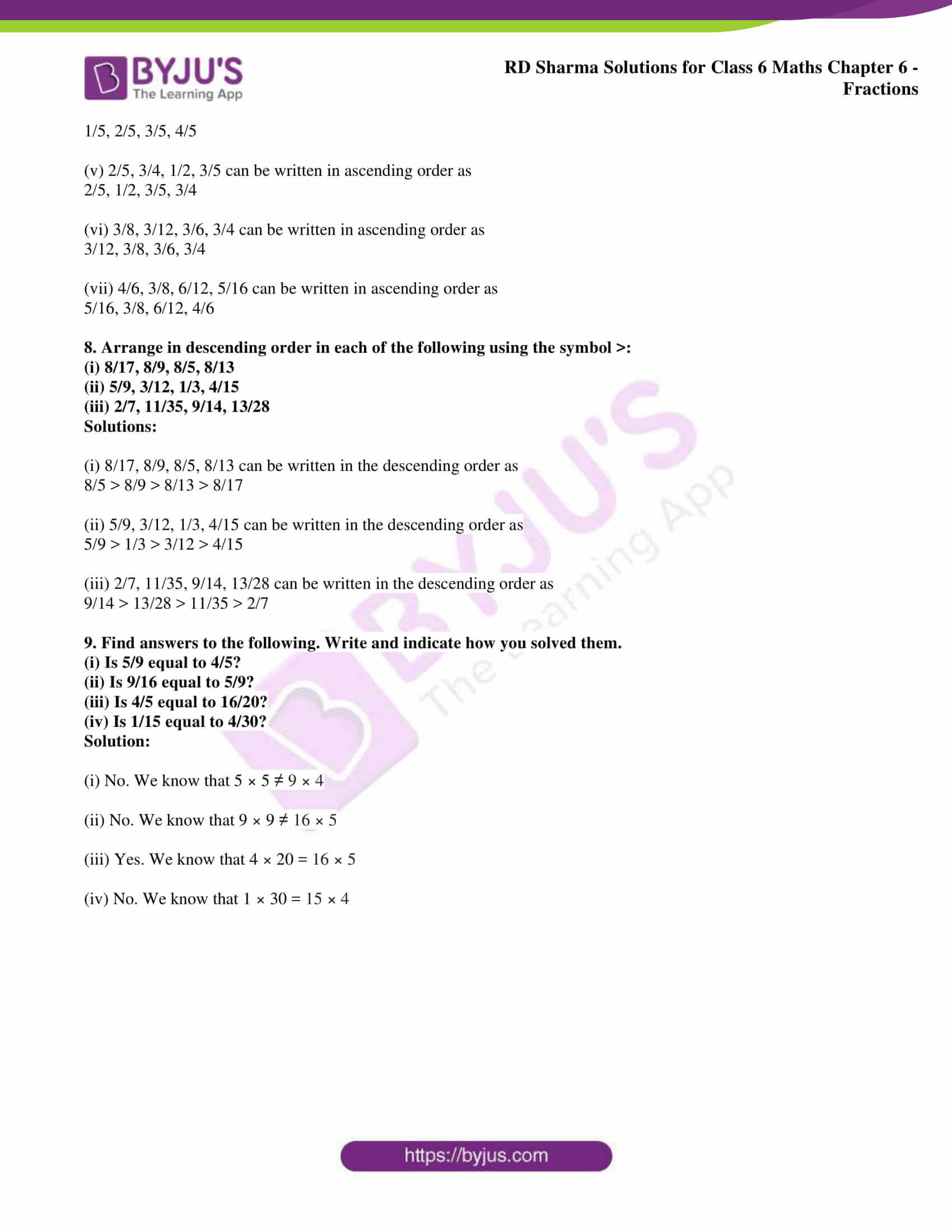### Access RD Sharma Solutions for Class 6 Chapter 6: Fractions Exercise 6.7

1. Write each fraction. Arrange them in ascending and descending order using correct sign <, =, > between the fractions: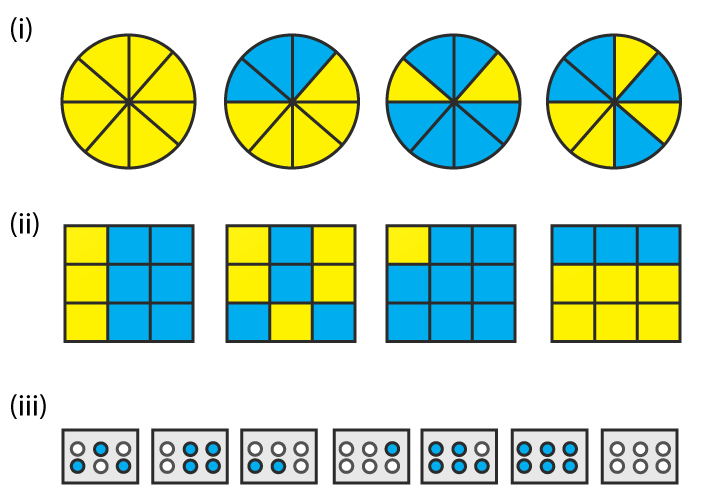Solution: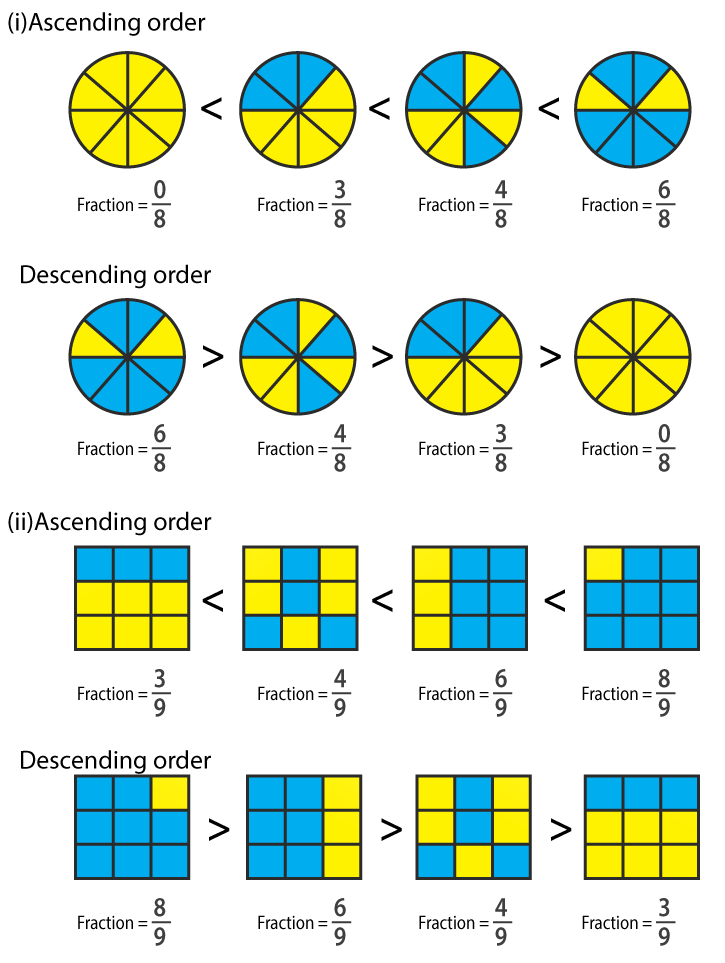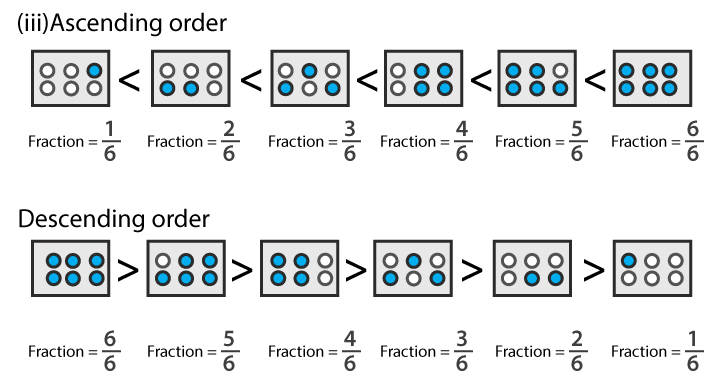2. Mark 2/6, 4/6, 8/6 and 6/6 on the number line and put appropriate signs between fractions given below:

(i) 5/6 …….. 2/6

(ii) 3/6 ……. 0/6

(iii) 1/6 …… 6/6

(iv) 8/6 …… 5/6

Solution: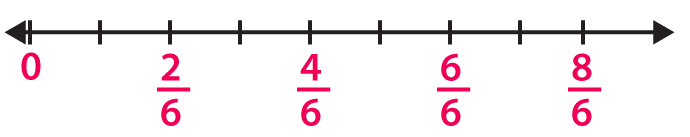(i) We know that

5/6 > 2/6 as 5 > 2 and the denominator is same.

(ii) We know that

3/6 > 0/6 as 3 > 0 and the denominator is same.

(iii) We know that

1/6 < 6/6 as 6 > 1 and the denominator is same.

(iv) We know that

8/6 > 5/6 as 8 > 5 and the denominator is same.

3. Compare the following fractions and put an appropriate sign:

(i) 3/6 …… 5/6

(ii) 4/5 …… 0/5

(iii) 3/20 …… 4/20

(iv) 1/7 ……. 1/4

Solution:

(i) We know that

3/6 < 5/6 as 3 < 5 and the denominator is same.

(ii) We know that

4/5 > 0/5 as 4 > 0 and the denominator is same.

(iii) We know that

3/20 < 4/20 as 3 < 4 and the denominator is same.

(iv) We know that

1/7 < 1/4 as 7 > 4 and the fraction having smaller denominator is larger.

4. Compare the following fractions using the symbol > or <:

(i) 6/7 and 6/11

(ii) 3/7 and 5/7

(iii) 2/3 and 8/12

(iv) 1/5 and 4/15

(v) 8/3 and 8/13

(vi) 4/9 and 15/8

Solution:

(i) We know that

6/7 > 6/11 as the fraction having smaller denominator is larger.

(ii) We know that

3/7 < 5/7 as 3 < 5 and the denominator is same.

(iii) We know that

8/12 = (2 × 2 × 2)/ (2 × 2 × 3) = 2/3

Hence, 2/3 = 8/12

(iv) We know that

1/5 = (1/ 5) × (3/3) = 3/15 which is lesser than 4/15

Hence, 1/5 < 4/15

(v) We know that

8/3 < 8/13 as the fraction having smaller value of denominator is larger.

(vi) We know that

4/9 = (4/9) × (8/8) = 32/72

15/8 = (15/8) × (9/9) = 135/72

So we get 32/72 < 135/72

Hence, 4/9 < 15/8.

5. The following fractions represent just three different numbers. Separate them in to three groups of equal fractions by changing each one to its simplest form:

(i) 2/12

(ii) 3/15

(iii) 8/50

(iv) 16/100

(v) 10/60

(vi) 15/75

(vii) 12/60

(viii) 16/96

(ix) 12/75

(x) 12/72

(xi) 3/18

(xii) 4/25

Solution:

(i) 2/12

We know that HCF of 2 and 12 = 2

By dividing numerator and denominator by HCF of 2 and 12

2/12 ÷ 2/2 = 1/6

(ii) 3/15

We know that HCF of 3 and 15 = 3

By dividing numerator and denominator by HCF of 3 and 15

3/15 ÷ 3/3 = 1/5

(iii) 8/50

We know that HCF of 8 and 50 = 2

By dividing numerator and denominator by HCF of 8 and 50

8/50 ÷ 2/2 = 4/25

(iv) 16/100

We know that HCF of 16 and 100 = 4

By dividing numerator and denominator by HCF of 16 and 100

16/100 ÷ 4/4 = 4/25

(v) 10/60

We know that HCF of 10 and 60 = 10

By dividing numerator and denominator by HCF of 10 and 60

10/60 ÷ 10/10 = 1/6

(vi) 15/75

We know that HCF of 15 and 75 = 15

By dividing numerator and denominator by HCF of 15 and 75

15/75 ÷ 15/15 = 1/5

(vii) 12/60

We know that HCF of 2 and 12 = 12

By dividing numerator and denominator by HCF of 2 and 12

12/60 ÷ 12/12 = 1/5

(viii) 16/96

We know that HCF of 16 and 96 = 16

By dividing numerator and denominator by HCF of 16 and 96

16/96 ÷ 16/16 = 1/6

(ix) 12/75

We know that HCF of 12 and 75 = 3

By dividing numerator and denominator by HCF of 12 and 75

12/75 ÷ 3/3 = 4/25

(x) 12/72

We know that HCF of 12 and 72 = 12

By dividing numerator and denominator by HCF of 12 and 72

12/72 ÷ 12/12 = 1/6

(xi) 3/18

We know that HCF of 3 and 18 = 3

By dividing numerator and denominator by HCF of 3 and 18

3/18 ÷ 3/3 = 1/6

(xii) 4/25

We know that HCF of 4 and 25 = 1

By dividing numerator and denominator by HCF of 4 and 25

4/25 ÷ 1/1 = 4/25

Three groups of equal fractions: 2/12, 10/60, 16/96, 12/72, 3/18, 3/15, 15/75, 12/60, 8/50, 16/100, 12/75, 4/25

6. Isha read 25 pages of a book containing 100 pages. Nagma read ½ of the same book. Who read less?

Solution:

No. of pages in the book = 100

We know that

Fraction of book Isha read = (25/100) ÷ (25/25) = 1/4 by dividing both numerator and denominator by HCF of 25 and 100

So the fraction of book Nagma read = 1/2

By comparing 1/4 and 1/2 we get the LCM of 4 and 2 = 4

Now convert the fraction into equivalent fraction having denominator as 4

1 × 1/4 × 1 and 1 × 2/2 × 2/14 and 1/4 = 2/4

7. Arrange the following fractions in the ascending order:

(i) 2/9, 7/9, 3/9, 4/9, 1/9, 6/9, 5/9

(ii) 7/8, 7/25, 7/11, 7/18, 7/10

(iii) 37/47, 37/50, 37/100, 37/1000, 37/85, 37/41

(iv) 3/5, 1/5, 4/5, 2/5

(v) 2/5, 3/4, 1/2, 3/5

(vi) 3/8, 3/12. 3/6, 3/4

(vii) 4/6, 3/8, 6/12, 5/16

Solution:

(i) 2/9, 7/9, 3/9, 4/9, 1/9, 6/9, 5/9 can be written in ascending order as

1/9, 2/9, 3/9, 4/9, 5/9, 6/9, 7/9

(ii) 7/8, 7/25, 7/11, 7/18, 7/10 can be written in ascending order as

7/25, 7/18, 7/11, 7/10, 7/8

(iii) 37/47, 37/50, 37/100, 37/1000, 37/85, 37/41 can be written in ascending order as

37/1000, 37/100, 37/85, 37/50, 37/47, 37/41

(iv) 3/5, 1/5, 4/5, 2/5 can be written in ascending order as

1/5, 2/5, 3/5, 4/5

(v) 2/5, 3/4, 1/2, 3/5 can be written in ascending order as

2/5, 1/2, 3/5, 3/4

(vi) 3/8, 3/12, 3/6, 3/4 can be written in ascending order as

3/12, 3/8, 3/6, 3/4

(vii) 4/6, 3/8, 6/12, 5/16 can be written in ascending order as

5/16, 3/8, 6/12, 4/6

8. Arrange in descending order in each of the following using the symbol >:

(i) 8/17, 8/9, 8/5, 8/13

(ii) 5/9, 3/12, 1/3, 4/15

(iii) 2/7, 11/35, 9/14, 13/28

Solutions:

(i) 8/17, 8/9, 8/5, 8/13 can be written in the descending order as

8/5 > 8/9 > 8/13 > 8/17

(ii) 5/9, 3/12, 1/3, 4/15 can be written in the descending order as

5/9 > 1/3 > 3/12 > 4/15

(iii) 2/7, 11/35, 9/14, 13/28 can be written in the descending order as

9/14 > 13/28 > 11/35 > 2/7

9. Find answers to the following. Write and indicate how you solved them.

(i) Is 5/9 equal to 4/5?

(ii) Is 9/16 equal to 5/9?

(iii) Is 4/5 equal to 16/20?

(iv) Is 1/15 equal to 4/30?

Solution:

(i) No. We know that 5 × 5 ≠ 9 × 4

(ii) No. We know that 9 × 9 ≠ 16 × 5

(iii) Yes. We know that 4 × 20 = 16 × 5

(iv) No. We know that 1 × 30 = 15 × 4